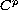Electron. J. Diff. Equ., Vol. 2010(2010), No. 74, pp. 1-14.

### A Holmgren type theorem for partial differential equations whose coefficients are Gevrey functions Masaki Kawagishi

Abstract:
In this article, we consider a uniqueness theorem of Holmgren type for p-th order Kovalevskaja linear partial differential equations whose coefficients are Gevrey functions. We prove that the only-solution to the zero initial-valued problem is the identically zero function. To prove this result we use the uniqueness theorem for higher-order ordinary differential equations in Banach scales.

Submitted March 28, 2010. Published May 21, 2010.
Math Subject Classifications: 35A05, 35A10, 35G10.
Key Words: Banach scale; Gevery function; Holmgren type; Uniqueness theorem.

Show me the PDF file (262 KB), TEX file, and other files for this article.Masaki Kawagishi Department of General Education College of Science and Technology, Nihon University 1-8-14 Kanda-Surugadai, Chiyoda-ku, Tokyo 101-8308, Japan email: masaki@suruga.ge.cst.nihon-u.ac.jp